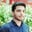Related Tags

dataframe
python
communitycreator

# How to concatenate text from multiple rows into a DataFrameSalman Yousaf

## Overview

The pandas library provides many functions for data manipulation. We can extract text from multiple rows using the groupby() method. The groupby() method performs the following:

• Divides the complete data into two sets according to their attributes.
• Extracts the string by the join function.
• Converts the values belonging to respective columns with the lambda function.

### Arguments

• The join() function: This function is used to get the string from the rows and columns.
• The lambda function: These anonymous functions do not require identification.
# import pandas library
import pandas as pd
# creating a DataFrame
df = pd.DataFrame([
(1, '2015', 10, 'A1'),
(2, '2015', 30, 'A2'),
(3, '2017', 35, 'A4'),
(4, '2022', 45, 'A2'),
(5, '2021', 44, 'A3'),
(6, '2020', 50, 'A3'),
], columns=['No.', 'Year', 'Marks', 'Assignment'])
# print DataFrame on console
print(df)
df['Assignment'] = df.groupby(['Year'])['Assignment'
].transform(lambda x: ' '.join(x))
# it will remove redundent entries
df = df.drop_duplicates()
# print updated DataFrame
print(df)

### Explanation

• Line 2: We import the pandas library as pd.
• Line 4–11: We define a DataFrame with four attributes and six entries.
• Line 14: We use df.groupby(['Year'])['Assignment'] to group ['Year'] values with df values. Next, the resultant returns the assignment column as ['Assignment'] is applied.
• Line 15: We use the transform(lambda x: ' '.join(x)) function to join exiting ['Assignment'] column to group by values. In pandas, transform() is used to produce self-generated values after applying it. The ' '.join(x) function adds a space character (' ') parallel to each value.
• Line 17–19: The df.drop_duplicates() function removes duplicate columns in specified DataFrame, df. Next, we print the DataFrame, df.

RELATED TAGS

dataframe
python
communitycreator

CONTRIBUTORSalman Yousaf
RELATED COURSES

View all Courses

Keep Exploring

Learn in-demand tech skills in half the time